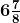# Use the distributivity of multiplication of rational numbers

Question:

Use the distributivity of multiplication of rational numbers over addition to simplify.

Solution:

(a) (3/5) × [(35/24) + (10/1)]

We know that the distributivity of multiplication of rational numbers over addition, a × (b + c) = a × b + a × c

Where, a =3/5, b =35/24, c = 10/1

Then, (3/5) × [(35/24) + (10/1)] = ((3/5) × (35/24)) + ((3/5) × (10/1))

= ((1/1) × (7/8)) + ((3/1) × (2/1))

= (7/8) + (6/1)

= (7 + 48)/8

= 55/8

=(b) (-5/4) × [(8/5) + (16/15)]

Solution:-

We know that the distributivity of multiplication of rational numbers over addition, a × (b + c) = a × b + a × c

Where, a =-5/4, b =8/5, c = 16/15

Then, (-5/4) × [(8/5) + (16/15)] = ((-5/4) × (8/5)) + ((-5/4) × (16/15))

= ((-1/1) × (2/1)) + ((-1/1) × (4/3))

= (-2/1) + (-4/3)

= (-6 – 4)/3

= -10/3

=-3 1/3

(c) (2/7) × [(7/16) – (21/4)]

Solution:-

We know that the distributivity of multiplication of rational numbers over subtraction, a × (b – c) = a × b – a × c

Where, a = -2/7, b = 7/16, c = 21/4

Then, (2/7) × [(7/16) – (21/4)] = ((2/7) × (7/16)) – ((2/7) × (21/4))

= ((1/1) × (1/8)) – ((1/1) × (3/2))

= (1/8) – (3/2)

= (1 – 12)/8

= -11/8

(d) ¾ × [(8/9) – 40]

Solution:-

We know that the distributivity of multiplication of rational numbers over subtraction, a × (b – c) = a × b – a × c

Where, a = -2/7, b = 7/16, c = 21/4

Then, (¾) × [(8/9) – (40)] = ((¾) × (8/9)) – ((¾) × (40))

= ((1/1) × (2/3)) – ((3/1) × (10))

= (2/3) – (30)

= (2 – 90)/3

= -88/3